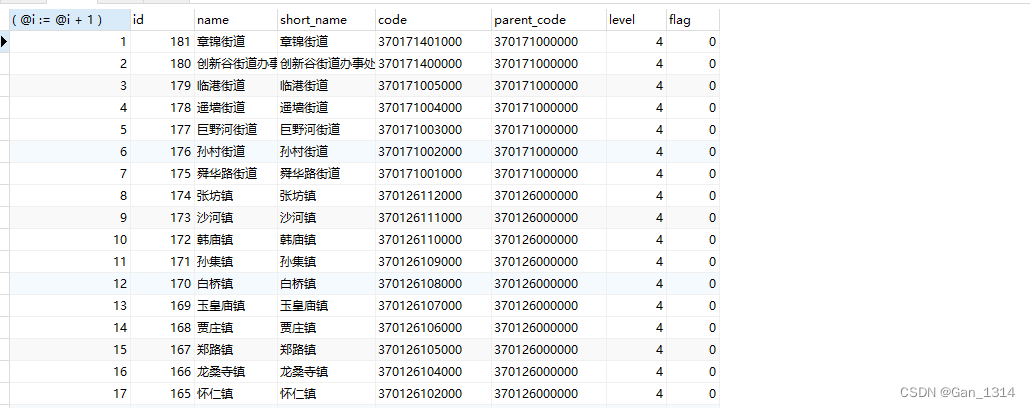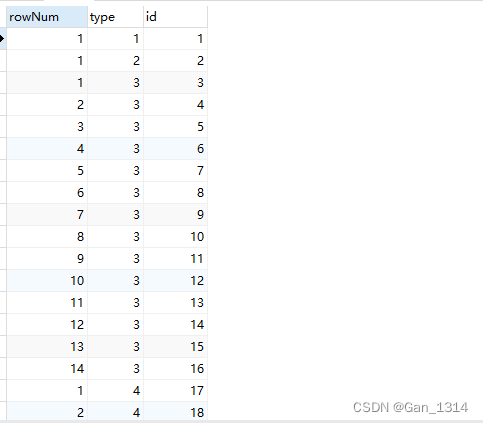# MySQL开发知识：MySQL中符号@的作用

`select @a;`

• @变量名 : 定义一个用户变量.
• = 对该用户变量进行赋值.

• 使用set命令对用户变量进行赋值时，两种方式都可以使用；
• 用select语句时，只能用”:=“方式，因为select语句中，”="号被看作是比较操作符。

(@i:=@i+1)

select (@i:=@i+5) as rownum, surname, personal_name from student, (select @i:=100) as init;

select @ids := 101,@l := 0

GROUP_CONCAT + group by

### mysql的@用法

1，增加临时表，实现变量的自增

SELECT (@i:=@i+1),t.* FROM table_name t,(SELECT @i:=0) AS j

(@i:=@i+1)代表定义一个变量，每次叠加1；
(SELECT @i:=0) AS j 代表建立一个临时表，j是随便取的表名，但别名一定要的。

2，实现排序递增

SELECT
( @i := @i + 1 ),
p.*
FROM
( SELECT * FROM sys_region ORDER BY create_time DESC ) p, ( SELECT @i := 0 ) k3，实现分组递增

SELECT
@r := CASE WHEN @type = a.LEVEL THEN
@r + 1
ELSE
1
END AS rowNum,
@type := a.`level` AS type,
a.id
FROM
sys_region a,( SELECT @r := 0, @type := ” ) b;### 实战

INSERT INTO t_top ( col_account, col_date, p_account, p_name )

SELECT b.col_account, b.col_date, b.p_account, b.p_name FROM
(
SELECT
@num := CASE WHEN @account = a.col_account THEN
@num + 1
ELSE
1
END AS rownum,
@account := a.col_account AS account,
a.*
FROM
( SELECT * FROM zb_top ORDER BY col_account, p_avg DESC ) a,
( SELECT @num := 0, @account := ” ) j
) b where 6 > b.rownum

CREATE TABLE `pd` (
`imei` varchar(32) NOT NULL DEFAULT ”,
`date` datetime DEFAULT NULL
) ENGINE=InnoDB DEFAULT CHARSET=utf8;

INSERT INTO `pd` VALUES (‘1’, ‘2013-07-25 00:00:01’);
INSERT INTO `pd` VALUES (‘1’, ‘2013-07-26 00:00:02’);
INSERT INTO `pd` VALUES (‘2’, ‘2013-07-23 00:00:04’);
INSERT INTO `pd` VALUES (‘2’, ‘2013-07-26 00:00:03’);
INSERT INTO `pd` VALUES (‘3’, ‘2013-07-26 00:00:01’);

SELECT * FROM
(
SELECT
imei user_id,
max(max_dd),
max(max_dd_2),
to_days(max(max_dd)) – to_days(max(max_dd_2)) days
FROM
(
SELECT
imei,
max_dd,
max_dd_2
FROM
(
SELECT
tmp.imei,
tmp.date,
IF(@imei = tmp.imei, @rank := @rank + 1, @rank := 1) AS ranks,
IF(@rank = 1, @max_d := tmp.date, @max_d := NULL) AS max_dd,
IF(@rank = 2, @max_d_2 := tmp.date, @max_d_2 := NULL) AS max_dd_2,
@imei := tmp.imei
FROM
(SELECT imei, date FROM pd ORDER BY imei ASC, date DESC) tmp,
(SELECT @rownum := 0, @imei := NULL, @rank := 0, @max_d := NULL, @max_d_2 := NULL) a
) result
) t
GROUP BY
imei
HAVING
count(*) > 1
) x WHERE x.days >= 1 AND EXISTS (SELECT ‘x’ FROM pd WHERE date > ‘2013-07-26 00:00:00’)